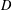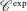Forum of Mathematics, Sigma ( IF 1.464 ) Pub Date : 2020-06-30 , DOI: 10.1017/fms.2020.27
AVRAHAM AIZENBUD; RAF CLUCKERS

Many phenomena in geometry and analysis can be explained via the theory of$D$ -modules, but this theory explains close to nothing in the non-archimedean case, by the absence of integration by parts. Hence there is a need to look for alternatives. A central example of a notion based on the theory of$D$ -modules is the notion of holonomic distributions. We study two recent alternatives of this notion in the context of distributions on non-archimedean local fields, namely$\mathscr{C}^{\text{exp}}$ -class distributions from Cluckers et al. [‘Distributions and wave front sets in the uniform nonarchimedean setting’, Trans. Lond. Math. Soc.5(1) (2018), 97–131] and WF-holonomicity from Aizenbud and Drinfeld [‘The wave front set of the Fourier transform of algebraic measures’, Israel J. Math.207(2) (2015), 527–580 (English)]. We answer a question from Aizenbud and Drinfeld [‘The wave front set of the Fourier transform of algebraic measures’, Israel J. Math.207(2) (2015), 527–580 (English)] by showing that each distribution of the$\mathscr{C}^{\text{exp}}$ -class is WF-holonomic and thus provides a framework of WF-holonomic distributions, which is stable under taking Fourier transforms. This is interesting because the$\mathscr{C}^{\text{exp}}$ -class contains many natural distributions, in particular, the distributions studied by Aizenbud and Drinfeld [‘The wave front set of the Fourier transform of algebraic measures’, Israel J. Math.207(2) (2015), 527–580 (English)]. We show also another stability result of this class, namely, one can regularize distributions without leaving the$\mathscr{C}^{\text{exp}}$ -class. We strengthen a link from Cluckers et al. [‘Distributions and wave front sets in the uniform nonarchimedean setting’, Trans. Lond. Math. Soc.5(1) (2018), 97–131] between zero loci and smooth loci for functions and distributions of the$\mathscr{C}^{\text{exp}}$ -class. A key ingredient is a new resolution result for subanalytic functions (by alterations), based on embedded resolution for analytic functions and model theory.

down
wechat
bug